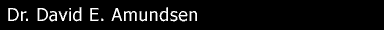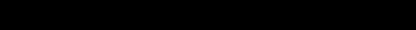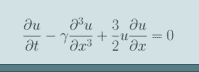Home Teaching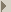MATH 5408/4708MATH 4907MATH 3008MATH 2404Putnam ResearchWhat is Applied MathematicsWhat I do Resources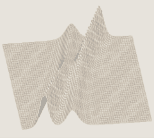# What Is Applied Mathematics

The term "Applied Mathematics" encompasses many different areas of mathematical research, and may be defined in numerous ways. Loosely speaking, it covers the intersection between mathematics and other scientific fields, such as physics, computer science, biology, engineering etc. with the driving motivation being a particular application or physical setting which researchers seek to understand more thoroughly. There is also often a distinction drawn between mathematics as it applies to problems with an underlying continuous structure (e.g. fluids) and problems with an underlying discrete structure (e.g. communication networks). The former typically draws more on techniques of analysis while the latter draws more on algebra. Nevertheless in either case is often found that understanding the mathematics behind one application leads to understanding of an entire class of mathematically similar, yet seemingly disparate physical settings.

My focus lies in continuous applications and to study such problems there are typically two key elements:

## 1) Modelling

The construction of a mathematical framework (equations) which approximate (rarely can we be exact) the application of interest. In many cases modelling is based on underlying physical conservation laws (conservation of mass, conservation of momentum etc.). However in general these in turn lead to very complicated systems of equations which can only be solved in very limited cases. It is therefore necessary to develop the models further, through techniques such as re-scaling and non-dimensionalization, and based on the particular setting at hand, decide which effects are "important" and which can be neglected. This depends not only on the effects themselves but the context in which they are being studied.

## 2) Analysis

Once a model is derived the next step is to explore its implications about the system's behaviour and where its limits of validity lie. Depending upon the complexity of the model, a mix of analytic and numerical (computer based) techniques may be used to accomplish this.

Typical analytic approaches include perturbation theory, asymptotic methods and dimensional analysis. A good survey can be found in MATH 4708/5408, Asymptotic Methods of Applied Mathematics. This course is appropriate, if not essential, for graduate and upper year undergraduate students who are looking to pursue further studies in applied mathematical fields. It is also open, accessible and potentially very useful to students from other disciplines in Science and Engineering with appropriate mathematical background. In general the key pre-requisites include an introductory course in ODEs (e.g. MATH 2454/2404) and Complex Analysis (e.g. MATH 3057/3007). This course is generally offered every other year, in alternation with MATH 5806 (see below).

Use of computational methodologies is another important tool which can be used to simulate and understand solutions of mathematical models. There are numerous techniques to choose from depending on the nature of the equations in the model and the physical domain in which they are to be studied. Interested students are directed to (at the undergraduate level) MATH 3806, MATH 4806 and (at the graduate level) MATH 5806.